## Subtracting mentally in Year 4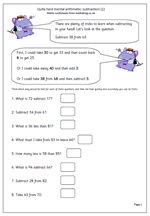Making the correct choice in deciding how to work out an answer is the key to fast mental arithmetic. Many children are unaware that there are often several ways of working out a calculation and that those who choose the best methods find maths easier and are able to answer questions more quickly and more accurately.

Let’s look at ‘Subtract 38 from 63.

This should be done, ‘in your head’ and will involve several stages, depending on the method used.

First, I could take 30 from 63 to leave 33. Then I could count back 8 from 33 which gives me 25.

Secondly, I could take 40 from 63 leaving 23 and then compensate by adding 2, which gives me 25.

Thirdly, I could take 38 from 68, leaving 30 and then compensate by subtracting 5 (because 63 is 5 less than 68).

There are several other ways very similar to these, but this does show that there is no ‘one right way, when it comes to mental arithmetic.

Quite hard mental arithmetic:_subtraction (1)

## Mental division practice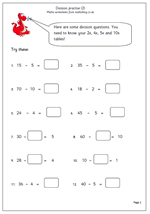being able to divide mentally is essential and is made much easier by knowing times tables.

Here is a follow up page to one published earlier, giving more practice with simple division. If children have a good knowledge of the 2x, 4x, 5x and 10x tables they should find these quite straightforward. The only potentially tricky ones are where the missing number is in the middle of the number sentence

eg 24 divided by ? = 6

This requires a good understanding of what the number sentence means, but all that is required to answer is a knowledge of ‘what multiplied by 6 makes 24’.

This worksheet can be found in our Year 3 Knowing Number Facts section.

Y3 division practice 2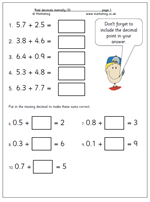Here is another page which looks at adding decimals mentally and once again shows the different approach which is usually taken compared to the standard written method.

To illustrate what I mean let’s look at the first question: 5.7 + 2.5. If this was done by the written method the two numbers would be placed in a vertical form:

5.7

2.5

and added starting with the tenths before going on to the units.

When using mental methods it would be quite usual to start with the units, adding the 5 and 2 to make 7, holding this in our memory, then adding the 7 and 5 tenths, making 1.2 before finally adding the 1.2and 7 to make 8.2.

The second set of questions on the page are probably best done by adding on. For example:

0.8 + ?? = 3

I would do this by adding 0.2 to 0.8 to make 1 and then counting on 2 more to make 3. There are , of course, other ways but the important thing is that whatever method is used it needs to be quick and accurate.

More of this type of question can be found in our Four Rules category.

## Pairs of numbers that make 20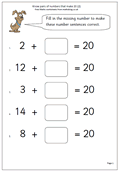Here we have a straightforward maths worksheet on knowing pairs of numbers that add up to twenty. This is suitable for year 1/2 children or those who are already very confident with knowing pairs of numbers that make ten.

If these facts are not known there are several ways of working the answers out, including:

1. Counting on from the smaller number.

2. Counting on from the smaller number up to 10 and then adding another 10. (If smaller number is below 10.)

3. Counting back from 20, which is trickier.

Know pairs that make 20 (pg 2)

## Roman numeral clock faces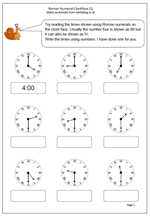One of the new targets for maths in year 3 will be to, ‘tell and write the time from an analogue clock, including using Roman numerals from I to XII’. As I haven’t published any time sheets using Roman numerals I thought that now was the perfect opportunity; so here it is!
It is best that children are introduced to the Roman system of numbers before doing this worksheet, although it is interesting to understand that many adults do not look at the numbers around the clock, just the angle of the two hands and indeed many fashion watches fail to have numbers on at all, but we still manage to be able to tell the time.

This page sticks to just whole hours and half hours, although perhaps it should be pointed out that Julius Caesar and his chums would never had read the time in this way!!

Roman numerals: clock faces

## Resource of the week: year 1 investigate additionWelcome to a new term and plenty of new maths material coming up over the next few months.

From our current resources here is a nice little investigation for young children which will show how well they can organise their thinking and work in a logical way.

The question is simple: how many different ways can you score 8 when throwing two dice?

It’s always a good idea to sit down with your children when doing this type of activity. In this way you can ask questions which will help them clarify their thoughts. The sheet is designed to be used as a record of results. At first they might just keep rolling the dice and adding up the totals until a total of 8 is achieved. Other children might dive straight in with some answers eg 4 + 4 makes 8.

A good question to ask at the end is “How do you know that you have got all the possibilities?

Free Y1 maths worksheet: Investigate dice

## Year 1 maths worksheet: numbers in order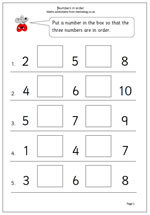I have just published a new worksheet on writing small numbers in order, for year 1 although suitable for younger children if ready. There is more than one possible answer on most of these which makes it a little trickier.
Many children learn to recite the numbers 1 to 10 without having any concept of what each number means so it is very important to show these numbers using practical ‘hands on’ equipment such as cubes, counters etc. and to understand one-to-one correspondence. To begin with some children will need to count up in ones from 1 to find each answer

There are also many activities which can help, such as tracing numbers in sand, tracing over numbers or using number cards to rearrange a small set of numbers in order.

Order numbers